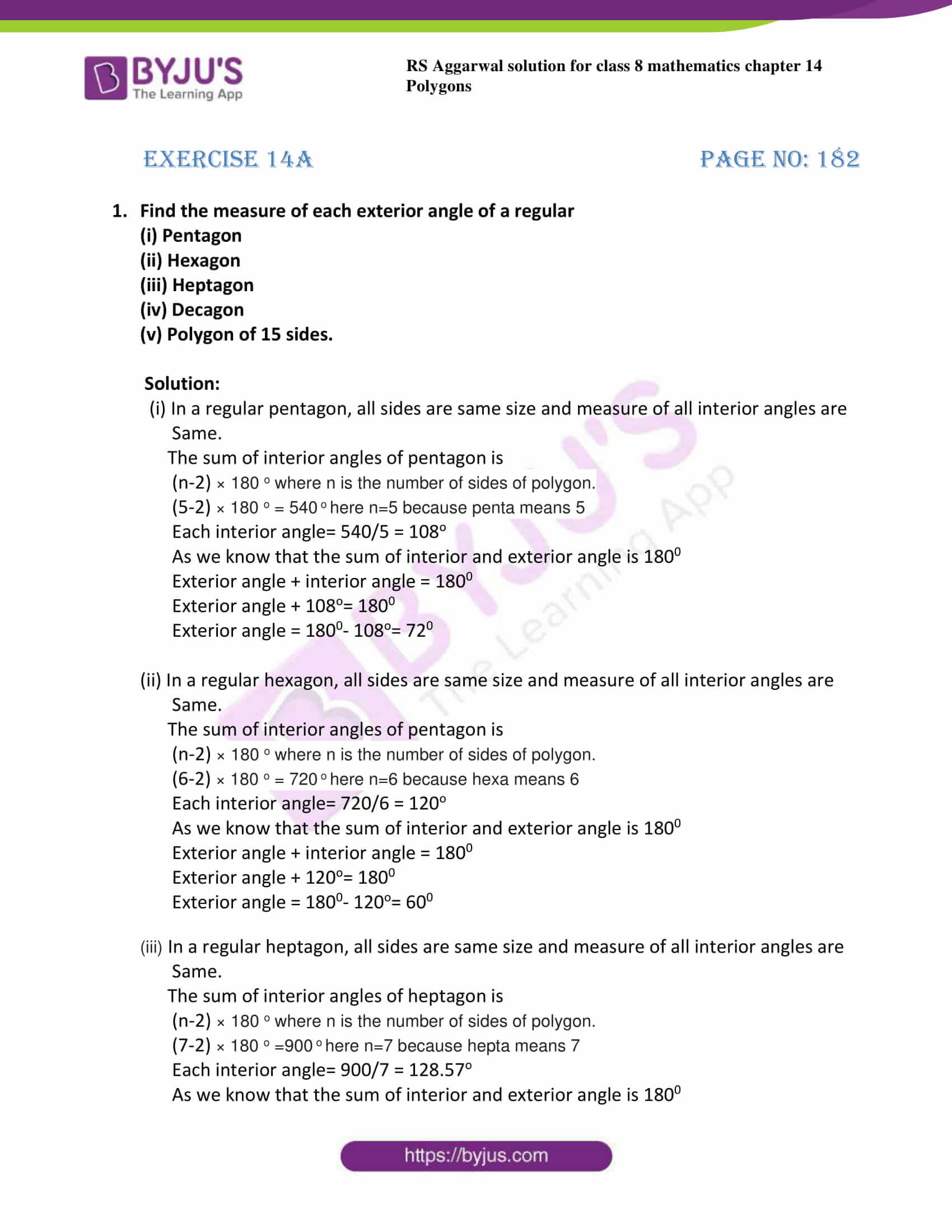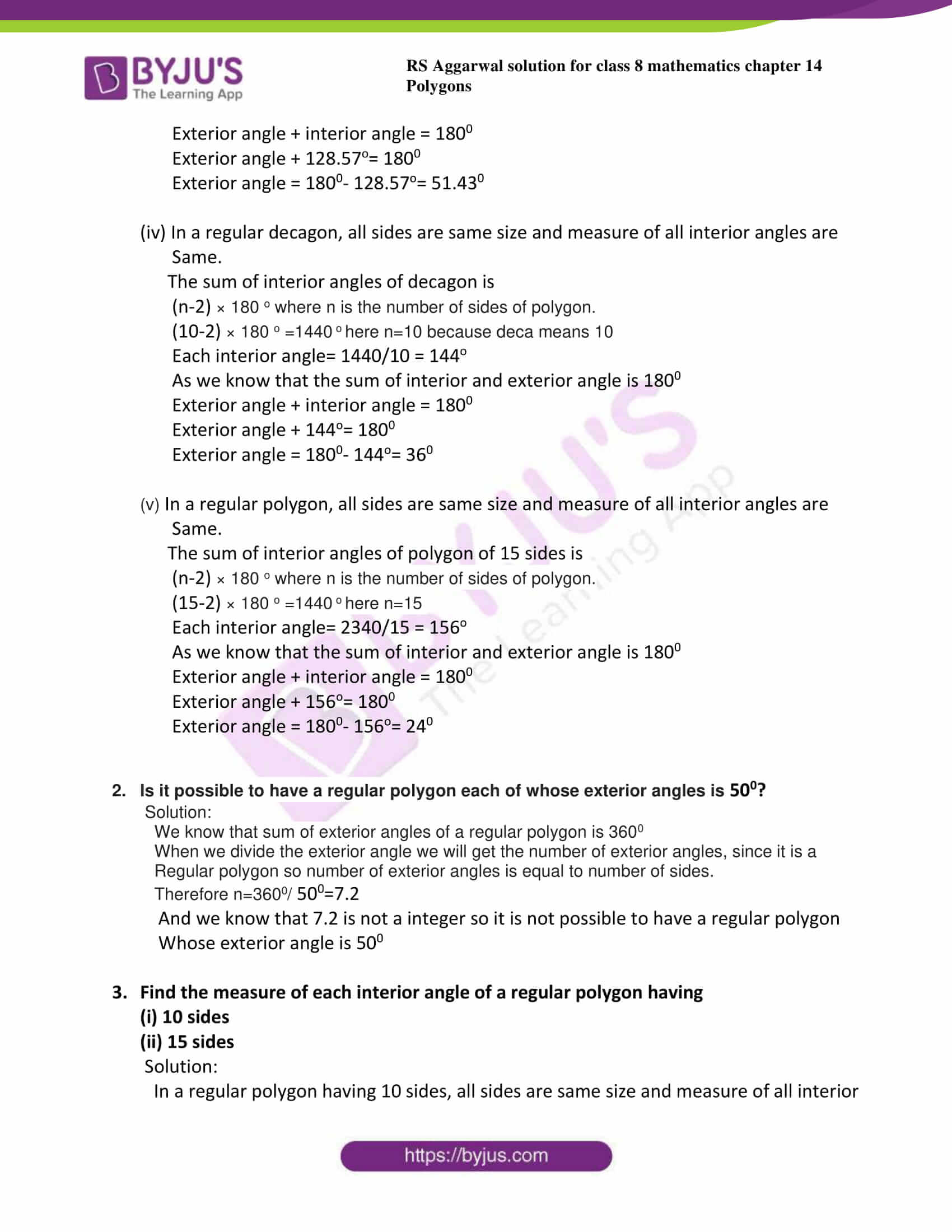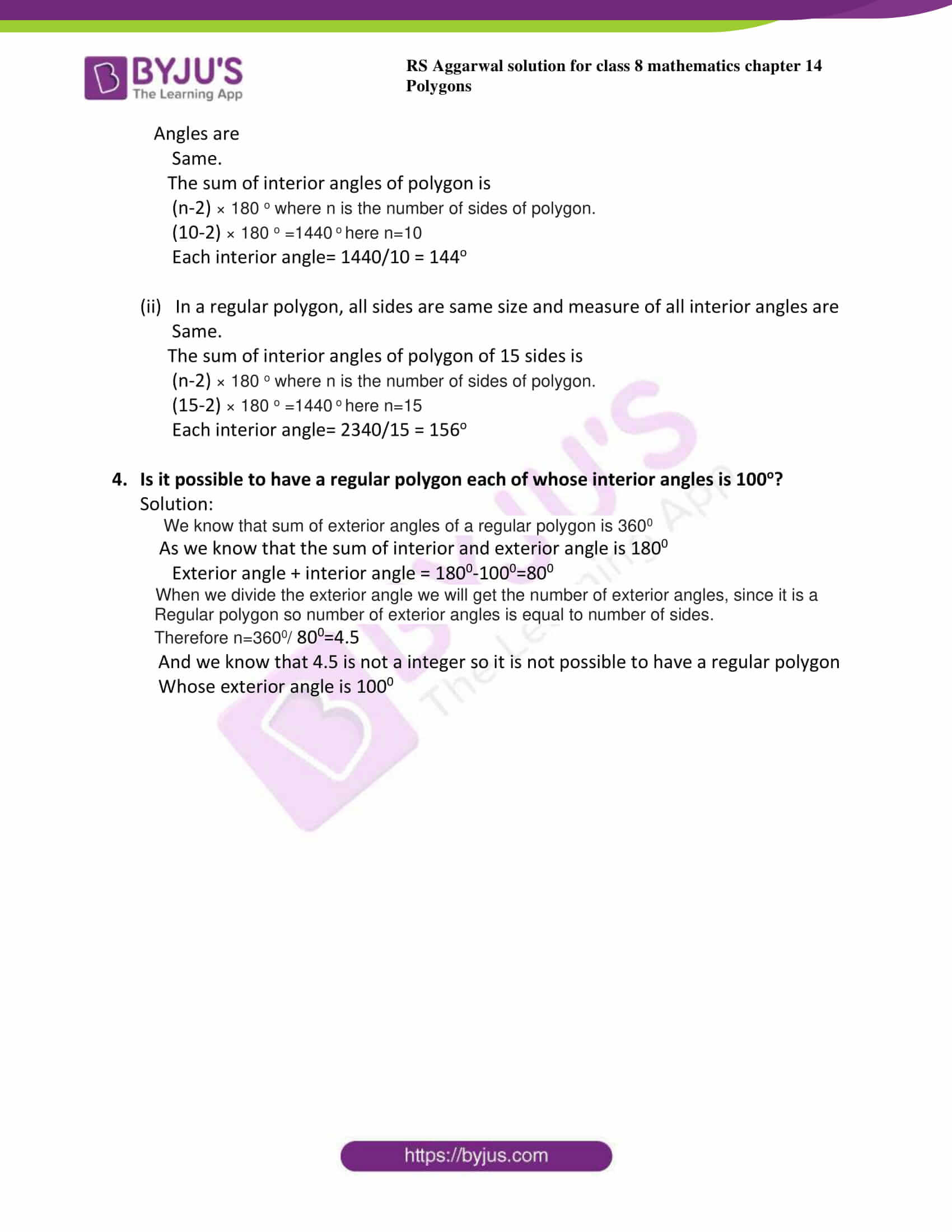# RS Aggarwal Solutions for Class 8 Maths Chapter 14 - Polygons Exercise 14A

RS Aggarwal Solutions for the Exercise 14A of Class 8 Maths Chapter 14, Polygons are available here. At BYJU’S, the subject experts solve the questions with the utmost care, giving proper knowledge to answer the questions. This exercise helps students to understand the concepts of polygons as well as curves, as this is the first exercise of this chapter, all problems based on basic concepts of polygons. By practising the RS Aggarwal Solutions for class 8, students will be able to grasp the concepts correctly. Hence, students whose aim is to score high in the examinations are advised to go through RS Aggarwal Solutions for Class 8.

## Download PDF of RS Aggarwal Solutions for Class 8 Maths Chapter 14 – Polygons Exercise 14A### Access answers to Maths RS Aggarwal Solutions for Class 8 Chapter 14 – Polygons Exercise 14A

1. Find the measure of each exterior angle of a regular

(i) Pentagon

(ii) Hexagon

(iii) Heptagon

(iv) Decagon

(v) Polygon of 15 sides.

Solution:

(i) In a regular pentagon, all sides are same size and measure of all interior angles are

Same.

The sum of interior angles of pentagon is

(n-2) × 180 o where n is the number of sides of polygon.

(5-2) × 180 o = 540 o here n=5 because penta means 5

Each interior angle= 540/5 = 108o

As we know that the sum of interior and exterior angle is 1800

Exterior angle + interior angle = 1800

Exterior angle + 108o= 1800

Exterior angle = 1800– 108o= 720

(ii) In a regular hexagon, all sides are same size and measure of all interior angles are

Same.

The sum of interior angles of pentagon is

(n-2) × 180 o where n is the number of sides of polygon.

(6-2) × 180 o = 720 o here n=6 because hexa means 6

Each interior angle= 720/6 = 120o

As we know that the sum of interior and exterior angle is 1800

Exterior angle + interior angle = 1800

Exterior angle + 120o= 1800

Exterior angle = 1800– 120o= 600

(iii) In a regular heptagon, all sides are same size and measure of all interior angles are

Same.

The sum of interior angles of heptagon is

(n-2) × 180 o where n is the number of sides of polygon.

(7-2) × 180 o =900 o here n=7 because hepta means 7

Each interior angle= 900/7 = 128.57o

As we know that the sum of interior and exterior angle is 1800

Exterior angle + interior angle = 1800

Exterior angle + 128.57o= 1800

Exterior angle = 1800– 128.57o= 51.430

(iv) In a regular decagon, all sides are same size and measure of all interior angles are

Same.

The sum of interior angles of decagon is

(n-2) × 180 o where n is the number of sides of polygon.

(10-2) × 180 o =1440 o here n=10 because deca means 10

Each interior angle= 1440/10 = 144o

As we know that the sum of interior and exterior angle is 1800

Exterior angle + interior angle = 1800

Exterior angle + 144o= 1800

Exterior angle = 1800– 144o= 360

(v) In a regular polygon, all sides are same size and measure of all interior angles are

Same.

The sum of interior angles of polygon of 15 sides is

(n-2) × 180 o where n is the number of sides of polygon.

(15-2) × 180 o =1440 o here n=15

Each interior angle= 2340/15 = 156o

As we know that the sum of interior and exterior angle is 1800

Exterior angle + interior angle = 1800

Exterior angle + 156o= 1800

Exterior angle = 1800– 156o= 240

2. Is it possible to have a regular polygon each of whose exterior angles is 500?

Solution:

We know that sum of exterior angles of a regular polygon is 3600

When we divide the exterior angle we will get the number of exterior angles, since it is a

Regular polygon so number of exterior angles is equal to number of sides.

Therefore n=3600/ 500=7.2

And we know that 7.2 is not a integer so it is not possible to have a regular polygon

Whose exterior angle is 500

3. Find the measure of each interior angle of a regular polygon having

(i) 10 sides

(ii) 15 sides

Solution:

In a regular polygon having 10 sides, all sides are same size and measure of all interior

Angles are

Same.

The sum of interior angles of polygon is

(n-2) × 180 o where n is the number of sides of polygon.

(10-2) × 180 o =1440 o here n=10

Each interior angle= 1440/10 = 144o

(ii) In a regular polygon, all sides are same size and measure of all interior angles are

Same.

The sum of interior angles of polygon of 15 sides is

(n-2) × 180 o where n is the number of sides of polygon.

(15-2) × 180 o =1440 o here n=15

Each interior angle= 2340/15 = 156o

4. Is it possible to have a regular polygon each of whose interior angles is 100o?

Solution:

We know that sum of exterior angles of a regular polygon is 3600

As we know that the sum of interior and exterior angle is 1800

Exterior angle + interior angle = 1800-1000=800

When we divide the exterior angle we will get the number of exterior angles, since it is a

Regular polygon so number of exterior angles is equal to number of sides.

Therefore n=3600/ 800=4.5

And we know that 4.5 is not a integer so it is not possible to have a regular polygon

Whose exterior angle is 1000

## RS Aggarwal Solutions for Class 8 Maths Chapter 14 – Polygons Exercise 14A

Exercise 14A of RS Aggarwal Solutions for Chapter 14, Polygons deals with the topics related to polygons and regular polygons. Some of the topics focused prior to exercise 14A include the following.

• Definitions of curve, open curve, closed curve
• Definition of polygons
• Definitions of a regular and irregular polygon

The RS Aggarwal Solutions can help the students in practising and learning each and every concept as it provides solutions to all questions asked in the RS Aggarwal textbook.